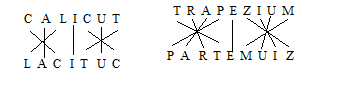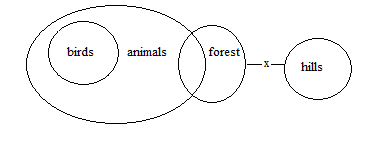# SSC CGL 2018 Practice Test Papers | Reasoning Ability (Day-11)

Dear Aspirants, Here we have given the Important SSC CGL Exam 2018 Practice Test Papers. Candidates those who are preparing for SSC CGL 2018 can practice these questions to get more confidence to Crack SSC CGL 2018 Examination.

[WpProQuiz 2801]

Click “Start Quiz” to attend these Questions and view Explanation

1). Select the related word/letters/ number from the given alternatives.

CALICUT: LACITUC::TRAPEZIUM: ?

a.PARTEMUIZ

b.PARTEZIUM

c.PTRAEZIUM

d.TRAPEMUIZ

2). Select the related word/letters/ number from the given alternatives.

73:37:: 25:??

1. 13
2. 20
3. 52
4. 10

3). Find out the odd word/letters/ number/ number pair from the given alternatives.

1. AC
2. KM
3. PR
4. JH

4). Find out the odd word/letters/ number/ number pair from the given alternatives.

1. 41-24
2. 60-34
3. 90-39
4. 80-54

5). A series is given with one term missing. Choose the correct alternative from the given ones that will complete the series

FDO, JHR, NLV,???

a. SVI

b.RZP

c.RPZ

d. SYO

6). A series is given with one term missing. Choose the correct alternative from the given ones that will complete the series.

45,56,69,?,105, 128

1. 100
2. 86
3. 76
4. 82

7). In the following question, select the missing number from the given series.

 7 9 64 5 8 42 10 4 ??
1. 50
2. 33
3. 47
4. 81
1. Statement:

All birds are animals.

Some animals are forest.

No forest is hills.

Conclusion

1. All Animals can never be Hills
2. No Hills is birds.
1. Only I follows
2. Only ii follows
3. Both I and ii follow
4. None of the above.

9). If “S” denotes “multiplied by”, “P” denotes “subtracted from”, “R” denotes “added to” and “Q” denotes “divided by”, then 121 Q 11 P 5 S 3 R 10 = ?

1. 23
2. 8
3. 6
4. 20

10.Arrange the given words in the sequence in which they occur in the dictionary.

1. causality
2. cause
3. categorize
1. 4312
2. 1234
3. 2143
4. 3421REVERSE OF 73-37

REVERSE OF 25-52

Except d all others are +2 series whereas JH is -2 series

41-24=17

60-26=34

90-39=51

80-54=26

Difference of two numbers are factors of 17 except d

45+11=56

56+13=6

69+17=86

86+19=105

105+23=128

(7+1)x(9-1)=64

(5+1)x(8-1)=42

(10+1)x(4-1)=33121/11-5×3+10=6

Correct order is